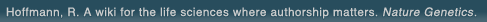# The world's first wiki where authorship really matters (Nature Genetics, 2008). Due credit and reputation for authors. Imagine a global collaborative knowledge base for original thoughts. Search thousands of articles and collaborate with scientists around the globe.

wikigene or wiki gene protein drug chemical gene disease author authorship tracking collaborative publishing evolutionary knowledge reputation system wiki2.0 global collaboration genes proteins drugs chemicals diseases compound
Hoffmann, R. A wiki for the life sciences where authorship matters. Nature Genetics (2008)# Study of pH-dependent zinc(II)-carboxamide interactions by zinc(II)-carboxamide-appended cyclen complexes (cyclen = 1,4,7,10-tetraazacyclododecane).

To elucidate intrinsic recognition of carboxamides by zinc(II) in carbonic anhydrase ( CA) (as inhibitors) and carboxypeptidase A ( CPA) (as substrates), a new series of Zn(2+)-carboxamide-appended cyclen complexes have been synthesized and characterized (cyclen = 1,4,7,10-tetraazacyclododecane). Two types of Zn(2+)-carboxamide interactions have been found. In the first case represented by a zinc(II) complex of carbamoylmethyl-1,4,7,10-tetraazacyclododecane (L(1)), the amide oxygen binds to zinc(II) at slightly acidic pH (to form ZnL(1)), and the deprotonated amide N(-) binds to zinc(II) at alkaline pH (to form ZnH(-1)L(1)) with pK(a) = 8.59 at 25 degrees C and I = 0.1 (NaNO(3)), as determined by potentiometric pH titrations, infrared spectral changes, and (13)C and (1)H NMR titrations. The X-ray crystal structure of ZnH(-1)L(3) (where L(3) = N-(4-nitrophenyl)carbamoylmethyl cyclen, pK(a) = 7.01 for ZnL(3) <==> ZnH(-1)L(3)) proved that the zinc(II) binds to the amidate N(-) (Zn-N(-) distance of 1.974(3) A) along with the four nitrogen atoms of cyclen (average Zn-N distance 2.136 A). Crystal data: monoclinic, space group P2(1)/n (No. 14) with a = 10.838(1) A, b = 17.210(2) A, c = 12.113(2) A, b = 107.38(1) degrees, V = 2156.2(5) A(3), Z = 4, R = 0.042, and R(w) = 0.038. These model studies provide the first chemical support that carboxamides are CA(-) inhibitors by occupying the active Zn(2+) site both in acidic and alkaline pH to prevent the occurrence of the catalytically active Zn(2+)-OH(-) species. In the second case represented by a zinc(II) complex of 1-(N-acetyl)aminoethylcyclen, ZnL(6), the pendant amide oxygen had little interaction with zinc(II) at acidic pH. At alkaline pH, the monodeprotonation yielded a zinc(II)-bound hydroxide species ZnL(6)(OH(-)) (pK(a) = 7.64) with the amide pendant remaining intact. The ZnL(6)(OH(-)) species showed the same nucleophilic activity as Zn(2+)-cyclen-OH(-). The second case may mimic the Zn(2+)-OH(-) mechanism of CPA, where the nucleophilic Zn(2+)-OH(-) species does not act as a base to deprotonate a proximate amide.